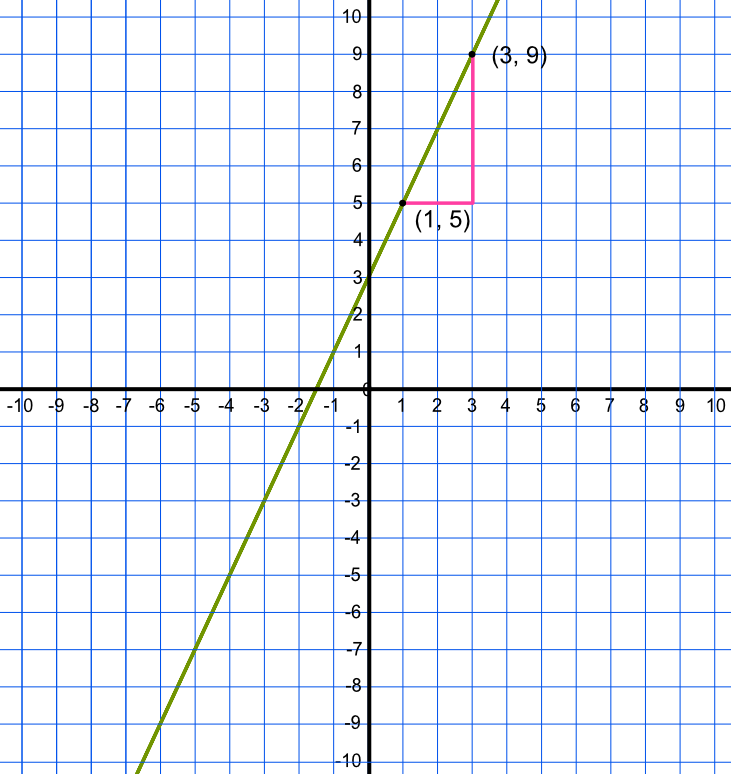Equation of a Line

# Equation of a Line

GCSE(F), GCSE(H),

Given two points on a straight line, the equation of the line can be found.

Use both points to work out the gradient; then substitute the values for one point into y = mx + c to obtain the intercept.

Given the points (1,5) and (3, 9): the gradient is given by frac("up")("along"), or frac("difference in y")("difference in x") = frac(9 - 5)(3 - 1) = frac(4)(2) = 2. The equation can now be written as y = 2x + c.

To work out the intercept, substitute the values for one of the points into the equation. Substituting the point (1, 5), the equation becomes 5 = 2 x 1 + c; therefore c = 3.

The equation is y = 2x + 3. Check using the second point.## Examples

1. Given the coordinates (3, 22) and (5, 32), determine the equation of the straight line.

Answer: y = 5x + 7

The difference between two points gives the gradient = frac("difference in y")("difference in x") = frac(32 - 22)(5 - 3) = 5.

Substitute in one point (3, 22) to obtain the intercept: 22 = 5 x 3 + c: c = 7. Check with the other point (5, 3): 32 = 5 x 5 + 7, so the answer is correct.

2. Given the coordinates (-3, 12.5) and (3, -11.5), determine the equation of the straight line.

Answer: y = -4x + 0.5

The difference between two points gives the gradient = frac("difference in y")("difference in x") = frac((-11.5 - 12.5))((3 - -3)) = frac(-24)(6) = -4.

Substitute in one point (-3, 12.5) to obtain the intercept: 12.5 = 4 x -3 + c: c = 0.5. Check with the other point (-11.5, 3): -11.5 = -4 x 3 + 0.5, so the answer is correct.